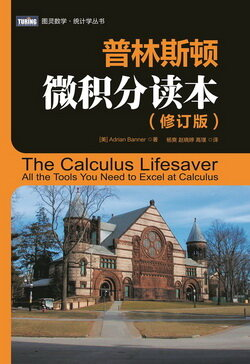# 普林斯顿微积分读本（修订版）(3)：函数、图像和直线 1.1.2(求定义域)

(1) 分数的分母不能是零.

(2) 不能取一个负数的平方根 (或四次根, 六次根, 等等).

(3) 不能取一个负数或零的对数. (还记得对数函数吗？若忘了, 请看看第 9 章!)

$\tan(90^\circ)=\frac{\sin(90^\circ)}{\cos(90^\circ)}=\frac{1}{0},$

tan(90°) 之所以是无定义的, 实际上是因为其隐藏的分母为零. 这里还有一个例子： 如果定义

$f(x)=\frac{\log_{10}(x+8)\sqrt{26-2x}}{(x-2)(x+19)},$

• 取 (26 - 2_x_) 的平方根, 所以这个量必须是非负的. 也就是说, 26 - 2_x_ ≥ 0. 这可以写成 x ≤ 13.
• 取 (x + 8) 的对数, 所以这个量必须是正的. (注意对数和平方根的区别：可以取 0 的平方根, 但不能取 0 的对数.) 不管怎么说, 我们需要 x + 8 > 0, 所以 x > -8. 到现在为止, 我们知道 -8 < x ≤ 13, 所以其定义域最多是 (-8, 13].
• 分母不能为 0, 这就是说 (x - 2) ≠ 0 且 (x + 19) ≠ 0. 换句话说, x ≠ 2 且 x ≠ -19. 最后一个条件不是问题, 因为我们已经知道 x 处于 (-8, 13] 内, 所以 x 不可能是 -19. 不过, 我们确实应该把 2 去掉.